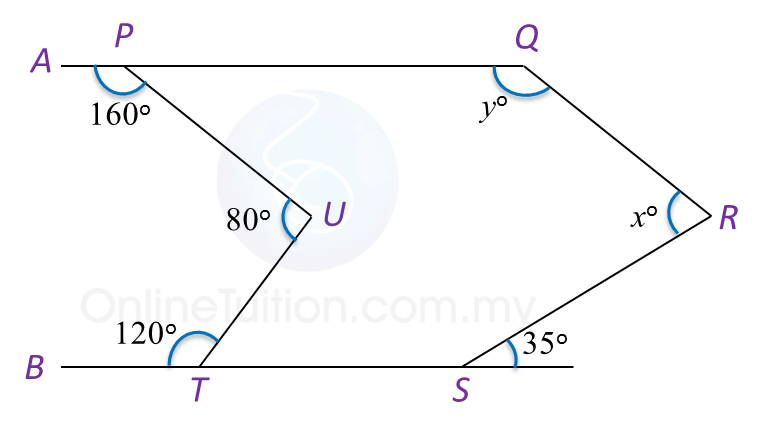# 4.2.1 Polygons, PT3 Practice

4.2.1 Polygons, PT3 Practice

Question 1:
Diagram below shows a pentagon PQRST. TPU and RSV are straight lines.Find the value of x.

Solution:

Question 2:
In Diagram below, PQRSTU is a hexagon. APQ and BTS are straight lines.Find the value of x + y.

Solution:

$\begin{array}{l}\angle QPU={180}^{o}-{160}^{o}={20}^{o}\\ \text{Reflex}\angle PUT={360}^{o}-{80}^{o}={280}^{o}\\ \angle UTS={180}^{o}-{120}^{o}={60}^{o}\\ \angle TSR={180}^{o}-{35}^{o}={145}^{o}\\ \\ \text{Sum of interior angles of a hexagon}\\ =\left(6-2\right)×{180}^{o}\\ ={720}^{o}\\ \\ {x}^{o}+{y}^{o}+{145}^{o}+{60}^{o}+{280}^{o}+{20}^{o}={720}^{o}\\ {x}^{o}+{y}^{o}={720}^{o}-{505}^{o}\\ \text{}={215}^{o}\\ \text{}x+y=215\end{array}$

Question 3:
Diagram below shows a regular hexagon PQRSTU. PUV is a straight line.Find the value of x + y.

Solution:
$\begin{array}{l}\text{Size of each interior angle of a regular hexagon}\\ =\frac{\left(6-2\right)×{180}^{o}}{6}\\ ={120}^{o}\\ {x}^{o}=\frac{{180}^{o}-{120}^{o}}{2}={30}^{o}\\ {y}^{o}={180}^{o}-{120}^{o}\\ \text{}={60}^{o}\\ {x}^{o}+{y}^{o}={30}^{o}+{60}^{o}\\ \text{}={90}^{o}\\ \text{}x+y=90\end{array}$

### 3 thoughts on “4.2.1 Polygons, PT3 Practice”

1.•2.